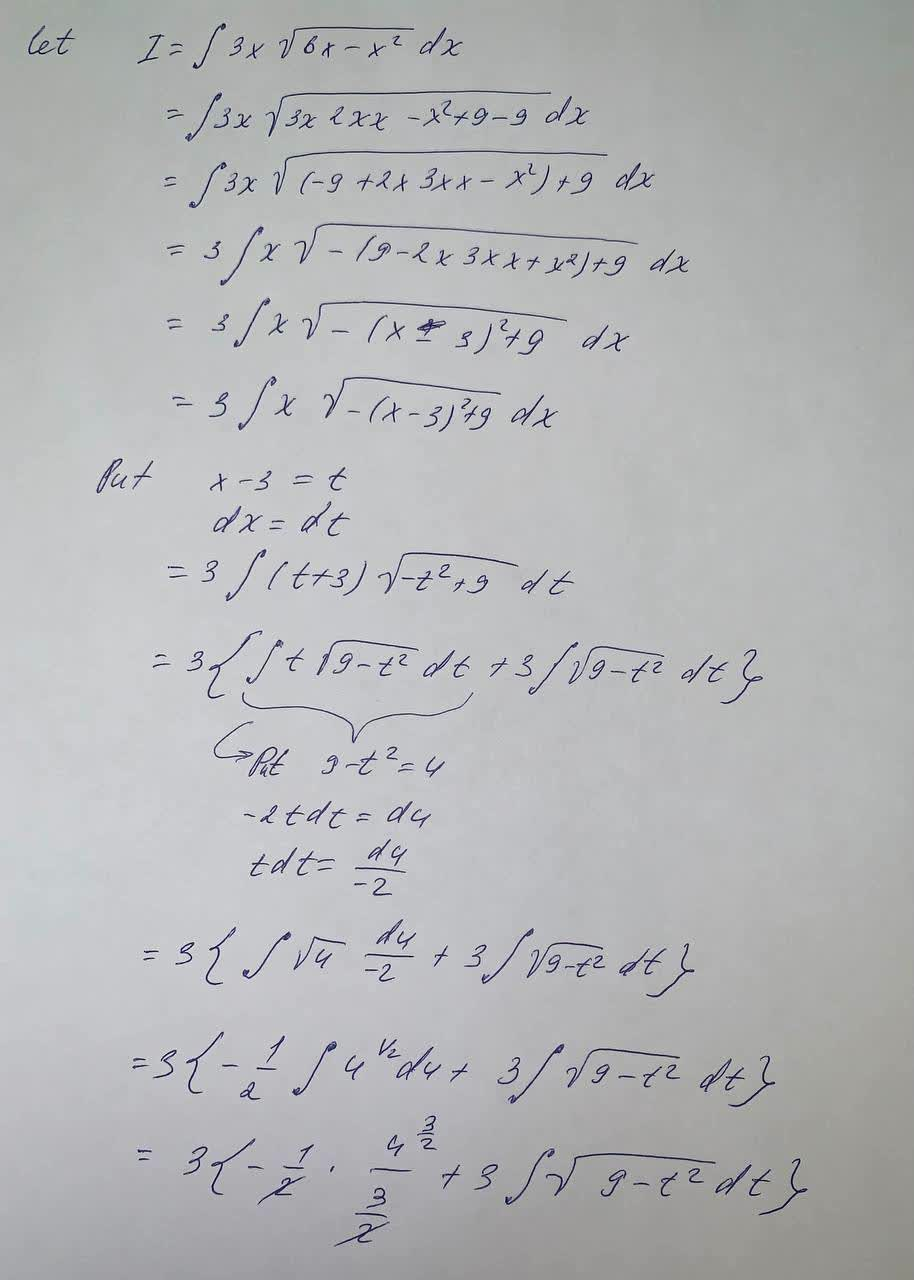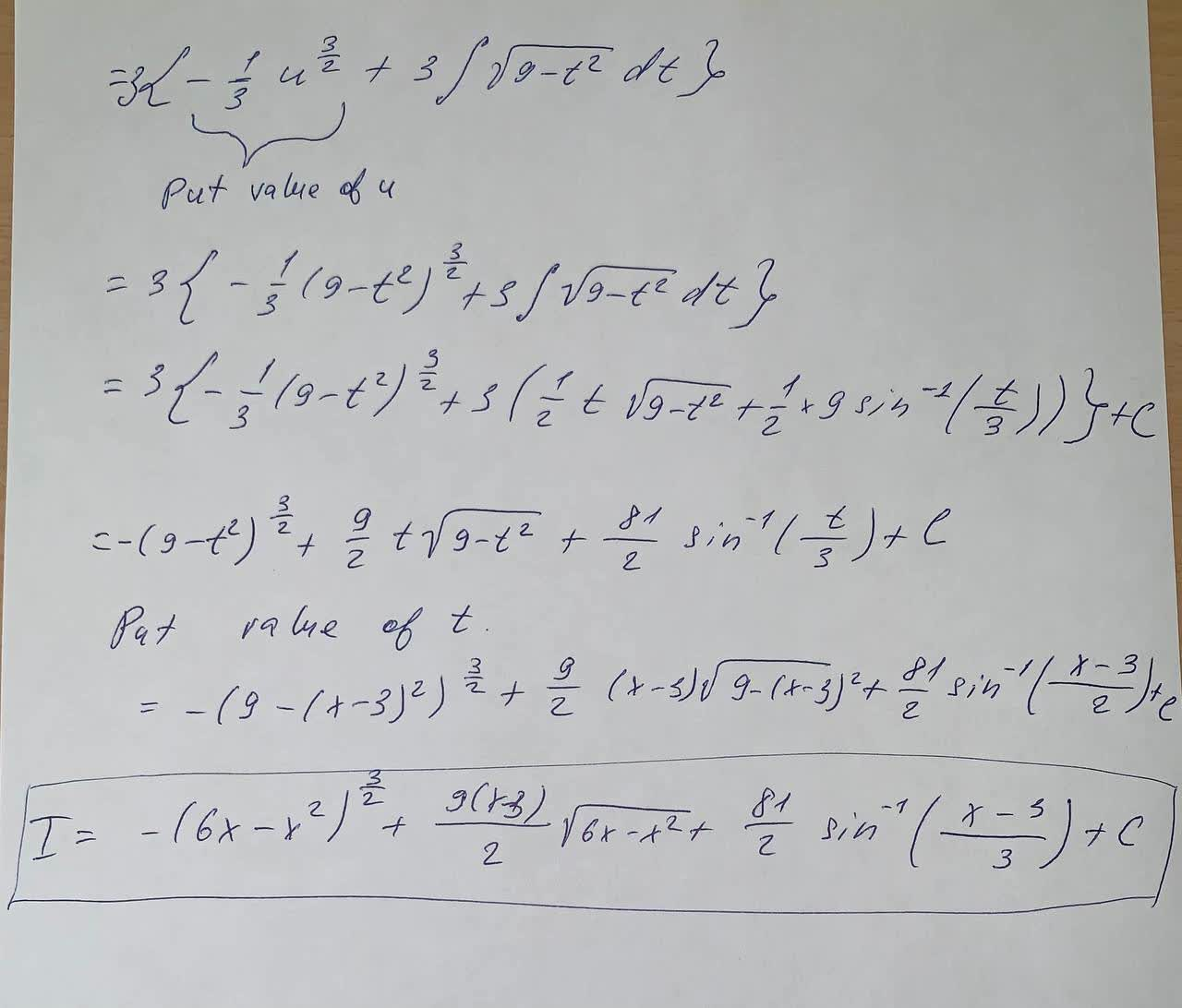Question# use the table of integrals to evaluate the following integral. \int 3x \sqrt{6x-x^2}dx

Applications of integrals
ANSWEREDuse the table of integrals to evaluate the following integral.
$$\int 3x \sqrt{6x-x^2}dx$$